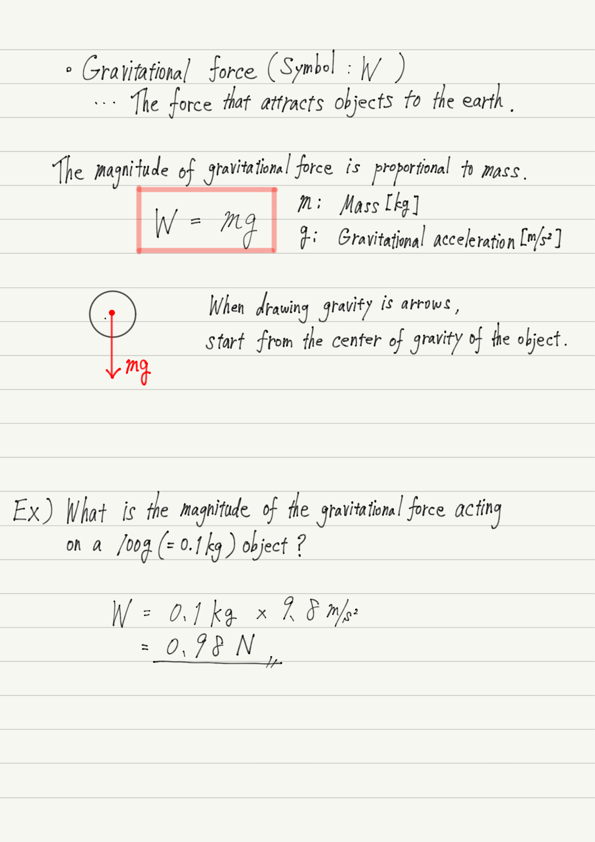# Gravitational Force

As announced in the previous lecture, let’s learn about various forces.
Top of the list is gravitational force!

Gravitational force, as we know, is the force that attracts an object toward the earth.

The goal of this lecture is to be able to calculate the magnitude of gravitational force.

## Magnitude of gravity

All objects on the earth are subject to gravity, but the magnitude of gravitational force is not constant.
The greater the mass of an object, the greater the gravitational force it receives.

To be precise, the magnitude of the gravitational force an object is subjected to is proportional to its mass.
The constant of proportionality is g (g is the gravitational acceleration. If you forgot, see the article below).

https://www.yukimura-physics.com/entry/dyn-f07

Therefore, the magnitude of gravitational force W can be expressed as W=mg using the mass of the object m and the gravitational acceleration g.

## Summary of this lecture## Next Time

In the next lecture, we will learn about the nature of tension force.

https://www.yukimura-physics.com/entry/dyn-f12

error: Content is protected !!